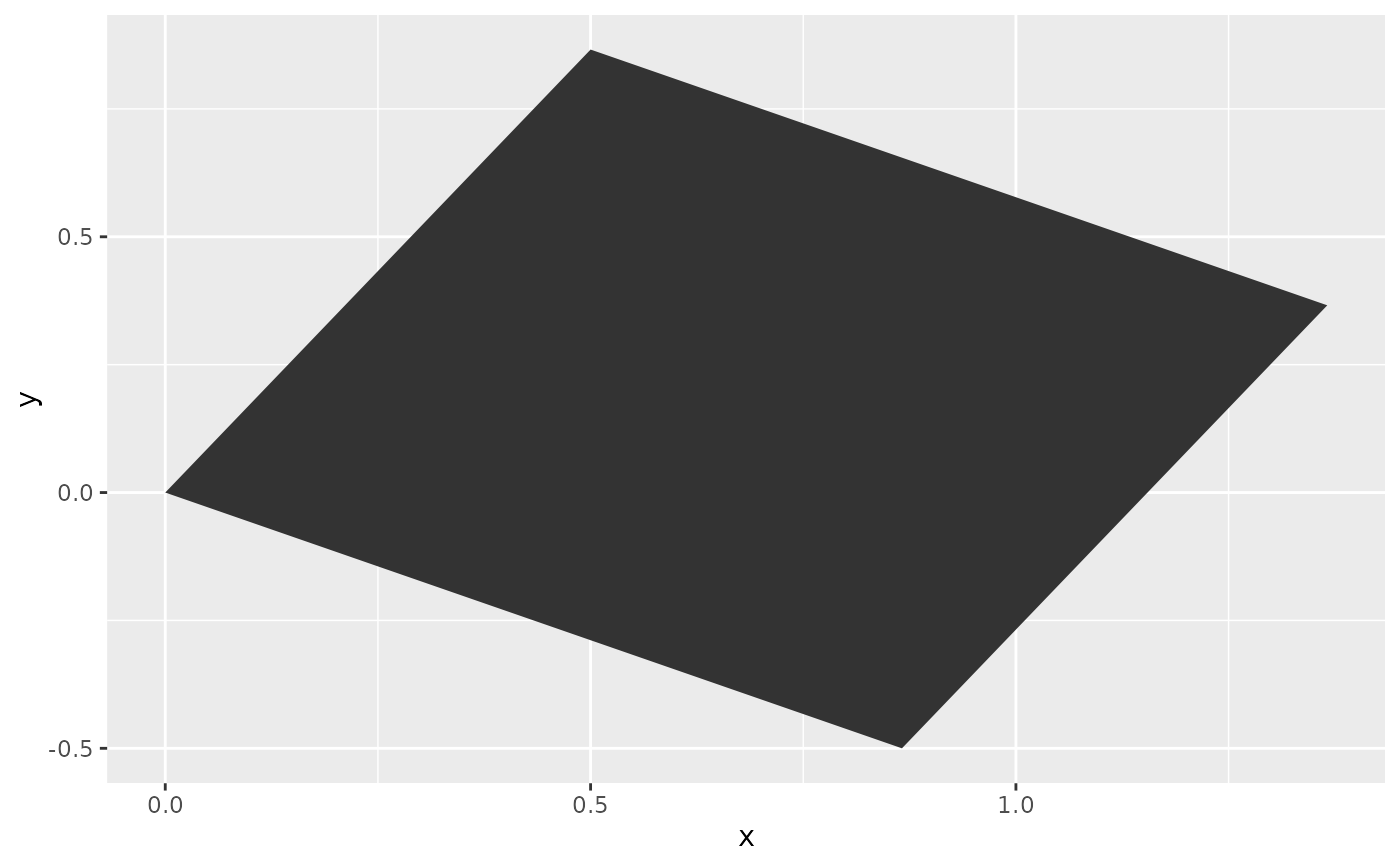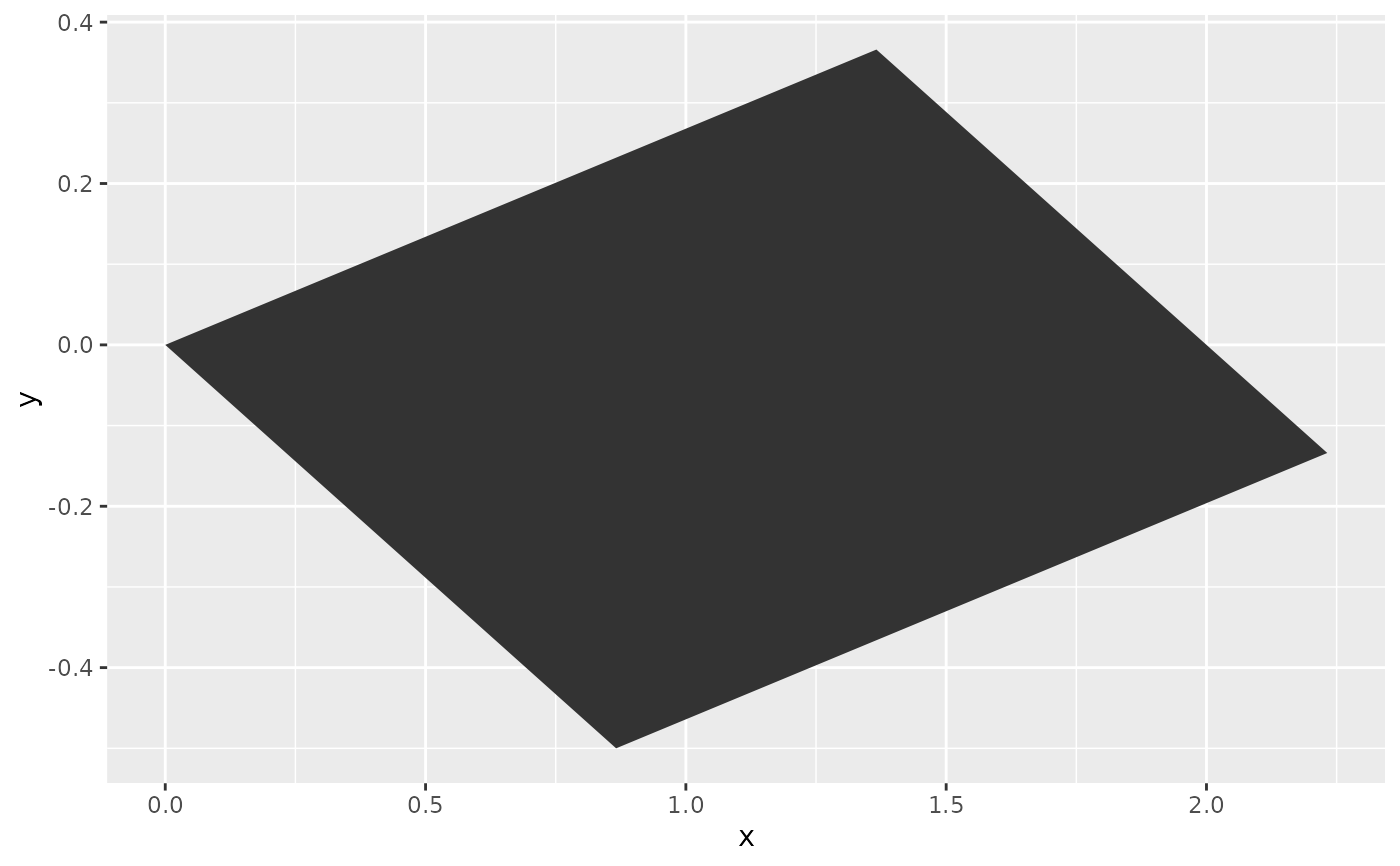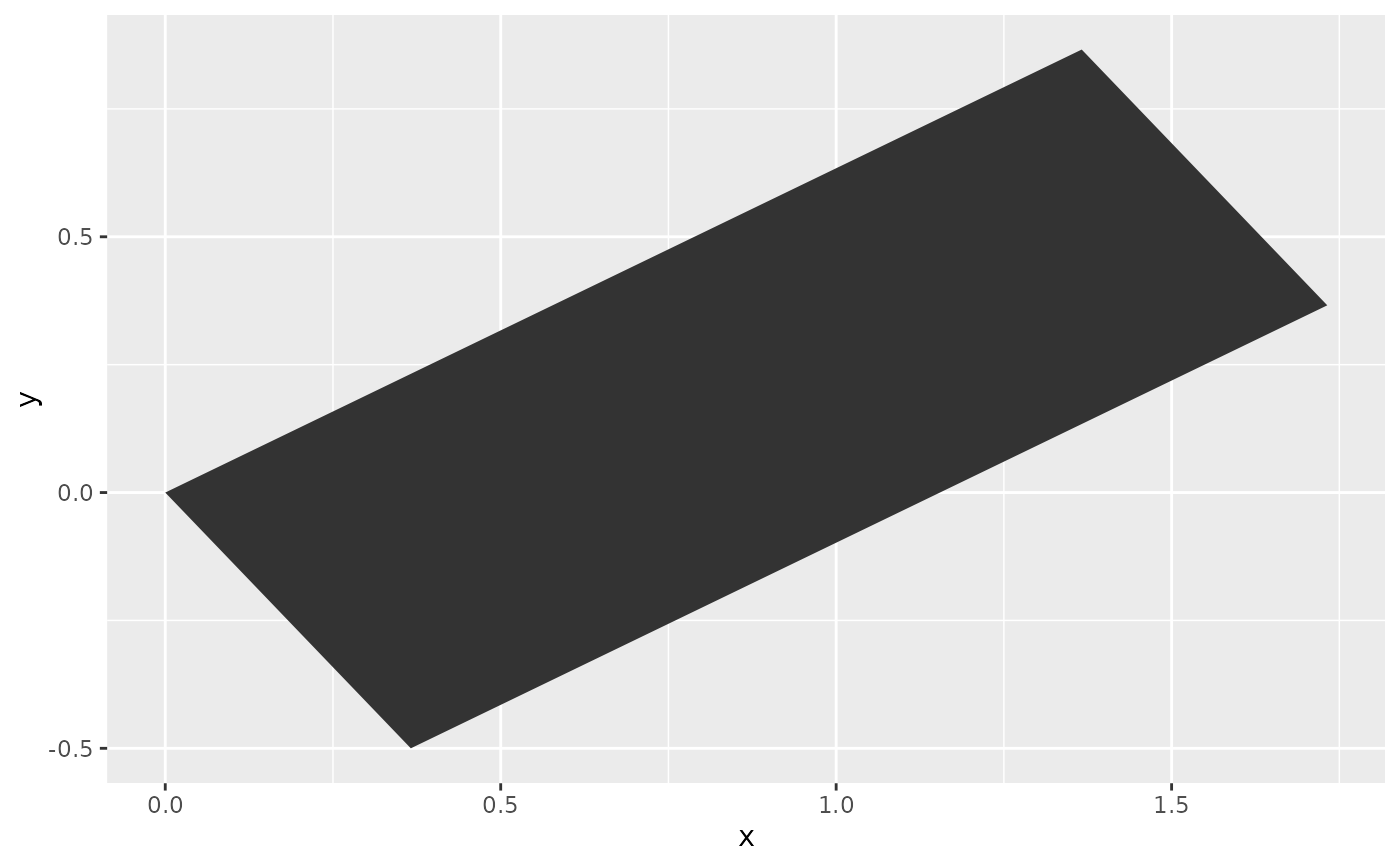Transforms coordinates in two dimensions in a linear manner for layers that have an x and y parametrisation.

## Usage

position_lineartrans(scale = c(1, 1), shear = c(0, 0), angle = 0, M = NULL)

## Arguments

scale

A numeric of length two describing relative units with which to multiply the x and y coordinates respectively.

shear

A numeric of length two giving relative units by which to shear the output. The first number is for vertical shearing whereas the second is for horizontal shearing.

angle

A numeric noting an angle in degrees by which to rotate the input clockwise.

M

A 2 x 2 real matrix: the transformation matrix for linear mapping. Overrides other arguments if provided.

## Value

A PositionLinearTrans ggproto object.

## Details

Linear transformation matrices are 2 x 2 real matrices. The 'scale', 'shear' and 'rotation' arguments are convenience arguments to construct a transformation matrix. These operations occur in the order: scaling - shearing - rotating. To apply the transformations in another order, build a custom 'M' argument.

For some common transformations, you can find appropriate matrices for the 'M' argument below.

## Common transformations

### Identity transformations

An identity transformation, or returning the original coordinates, can be performed by using the following transformation matrix:

| 1 0 |
| 0 1 |

or

M <- matrix(c(1, 0, 0, 1), 2)

### Scaling

A scaling transformation multiplies the dimension of an object by some amount. An example transformation matrix for scaling everything by a factor 2:

| 2 0 |
| 0 2 |

or

M <- matrix(c(2, 0, 0, 2), 2)

### Squeezing

Similar to scaling, squeezing multiplies the dimensions by some amount that is unequal for the x and y coordinates. For example, squeezing y by half and expanding x by two:

 | 2 0 | | 0 0.5 |

or

M <- matrix(c(2, 0, 0, 0.5), 2)

### Reflection

Mirroring the coordinates around one of the axes. Reflecting around the x-axis:

 | 1 0 | | 0 -1 |

or

M <- matrix(c(1, 0, 0, -1), 2)

Reflecting around the y-axis:

 | -1 0 | | 0 1 |

or

M <- matrix(c(-1, 0, 0, 1), 2)

### Projection

For projecting the coordinates on one of the axes, while collapsing everything from the other axis. Projecting onto the y-axis:

 | 0 0 | | 0 1 |

or

M <- matrix(c(0, 0, 0, 1), 2)

Projecting onto the x-axis:

 | 1 0 | | 0 0 |

or

M <- matrix(c(1, 0, 0, 0), 2)

### Shearing

Tilting the coordinates horizontally or vertically. Shearing vertically by 10\

 | 1 0 | | 0.1 1 |

or

M <- matrix(c(1, 0.1, 0, 1), 2)

Shearing horizontally by 200\

 | 1 2 | | 0 1 |

or

M <- matrix(c(1, 0, 2, 1), 2)

### Rotation

A rotation performs a motion around a fixed point, typically the origin the coordinate system. To rotate the coordinates by 90 degrees counter-clockwise:

 | 0 -1 | | 1 0 |

or

M <- matrix(c(0, 1, -1, 0), 2)

For a rotation around any angle $$\theta$$ :

 | $$cos\theta$$ $$-sin\theta$$ | | $$sin\theta$$ $$cos\theta$$ |

or

M <- matrix(c(cos(theta), sin(theta), -sin(theta), cos(theta)), 2)
with 'theta' defined in radians.

## Examples

df <- data.frame(x = c(0, 1, 1, 0),
y = c(0, 0, 1, 1))
ggplot(df, aes(x, y)) +
geom_polygon(position = position_lineartrans(angle = 30))# Custom transformation matrices
# Rotation
theta <- -30 * pi / 180
rot <- matrix(c(cos(theta), sin(theta), -sin(theta), cos(theta)), 2)
# Shear
shear <- matrix(c(1, 0, 1, 1), 2)

# Shear and then rotate
M <- rot %*% shear
ggplot(df, aes(x, y)) +
geom_polygon(position = position_lineartrans(M = M))# Alternative shear and then rotate
ggplot(df, aes(x, y)) +
geom_polygon(position = position_lineartrans(shear = c(0, 1), angle = 30))# Rotate and then shear
M <- shear %*% rot
ggplot(df, aes(x, y)) +
geom_polygon(position = position_lineartrans(M = M))